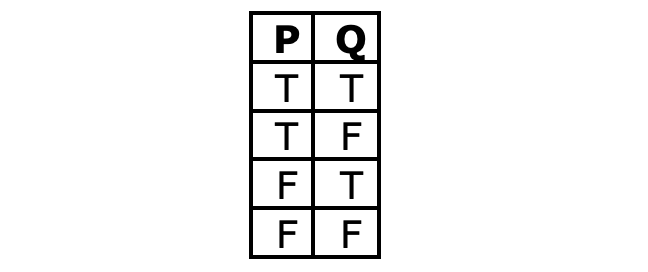## Want to keep learning?

This content is taken from the University of York's online course, Logic: The Language of Truth. Join the course to learn more.
4.11

## University of York# The problem, and the solution

## The problem

Remember when we first introduced the idea of deductive validity and started looking for counterexamples to particular arguments and forms of argument. There we simply used our imaginations in an unsystematic way. And we noted an issue with this …

If we found a counterexample to a form of argument, we could conclude with confidence that the form was invalid. If, on the other hand, we failed to find a counterexample, while that provided some reason to think the argument was valid, we saw that it would be less than fully conclusive, because it might be that we’d simply overlooked a counterexample. We have a conclusive negative test for validity, but not yet a conclusive positive test for validity—a test where, if an argument passes it, we can be confident that it is valid.

We’re in a position to provide a solution to this problem, at least for the argument forms of propositional logic, where the validity or invalidity of an argument depends on how it is made up from sentential clauses and truth-functional sentence-connectives.

## The solution

The key to the solution lies in the nature of truth-tables. (You might have spotted this already. We’ve implicitly appealed to it at a number of points.)

When we construct a truth-table relating to some particular basic sentences, we can and we do ensure that we produce a table which has rows corresponding to every possible way that things could be with regard to those sentences in terms of truth and falsity. For example, if we’re considering two basic sentences ‘P’ and ‘Q’, we know that there are four ways things could be with them in terms of truth and falsity, corresponding to the four rows of the following truth-table:#### Figure 1. Truth-table listing all possible combinations of truth-values for two basic sentences

(If we’re looking at arguments with larger numbers of basic sentences, we’d need to have more rows. As we saw earlier, if there are three basic sentences, we’d need eight rows; with four, we’d need 16; and so on, doubling the number with each additional basic sentence.)

The fact that a truth-table lists all of the relevant kinds of possible situation gives us a simple way of conducting an effective positive test for formal validity in propositional logic: construct a truth-table; work out the truth-values of the premises and conclusion on each row; then look to see if there are any rows on which all of the premises are true and the conclusion false.

If there are any such rows, the argument is invalid. And crucially, if there are not, we can conclude with confidence that the argument is formally valid, because we will know that we have looked everywhere that a counterexample might have lurked—we know we will have considered every relevant type of possible situation.

Let’s see how this works with a simple example.